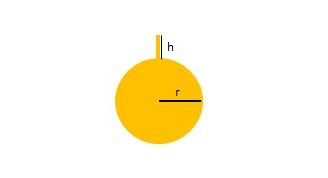Consider the following depiction of a tank with radius r= 6 m with a spout of height h= 2m. If...

Question:

Consider the following depiction of a tank with radius r= 6 m with a spout of height h= 2m. If the tank is half full of oil that has a density of 900 {eq}kg/m^3 {/eq}. Find the work W required to pump the oil out of the spout. Use 9.8 {eq}m/s^2 {/eq} for g and 3.14 for {eq}\pi {/eq}.Work required to pump oil in a spherical tank

In this problem a spherical tank is half-filled with oil. To pump the oil by doing work, a force that is equal to the weight of the oil must be exerted over a certain displacement. Here the weight of the oil will be computed from its density and the volume of the sphere and the displacement will be the diameter of the sphere plus the spout height.

• {eq}\displaystyle \text{radius} = r = 6 \,\mathrm{m} {/eq}
• {eq}\displaystyle \text{spout height} = h = 2 \,\mathrm{m} {/eq}
• {eq}\displaystyle \text{density of oil} = \rho = 900 \,\mathrm{kg/m^3} {/eq}
• {eq}\displaystyle \text{acceleration due to gravity} = g = 9.8 \,\mathrm{m/s^2} {/eq}
• {eq}\displaystyle \text{pi} = \pi = 3.14 {/eq}

The formula for work is,

{eq}\displaystyle W = Fd {/eq}

where F is the force exerted and d is the displacement. Here the displacement will be the height of the sphere which is the diameter (twice the radius) plus the height of the spout.

{eq}\displaystyle \text{displacement} = d = 12 + 2 = 14 \,\mathrm{m} {/eq}

The weight of water is equivalent to the F,

{eq}\displaystyle F = \text{weight of water} {/eq}

The formula for the weight,

{eq}\displaystyle \text{weight} = \rho V g {/eq}

where V is the volume of the water needed to be displaced.

The formula for volume is,

{eq}\displaystyle V = \frac{4}{3}\pi r^3 {/eq}

Plug-in all the expression into the formula for work,

{eq}\displaystyle W = \rho\frac{4}{3}\pi r^3 g d {/eq}

plug-in all the values,

{eq}\displaystyle W = (900 \,\mathrm{kg/m^3})\frac{4}{3} (3.14) (6 \,\mathrm{m} )^3 (9.8 \,\mathrm{m/s^2} )(14 \,\mathrm{m}) {/eq}

multiply and cancel units,

{eq}\displaystyle W = 111,665 \,\mathrm{kg \cdot m^2/s^2} = 111,665 \,\mathrm{J} {/eq}

Note that,

{eq}\displaystyle 1\,\mathrm{N} = 1 \,\mathrm{kg \cdot m/s^2} {/eq}

and

{eq}\displaystyle 1\,\mathrm{N*m} = 1 \,\mathrm{J} {/eq}

The amount of work required to pump the water out is {eq}\displaystyle 111,665 \,\mathrm{J} {/eq}.